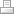PaulsenConsult.com

# Cash Flow from Operating Activities

We will now design our own Cash Flow Statement Account Schedule CASHFLOWST.

The next picture shows the Operating Activities section of the Cash Flow Statement:

Net Income

The Operating section of the Cash Flow Statement starts with Row R01 Net Income.

In the Chart of Accounts, you can see the very last G/L Account 99999 NET INCOME is defined as the Total for all Income Statement G/L Accounts 40000..99999:

Dynamics NAV records revenues as negative amounts and expenses as positive amounts. In the above picture a date filter for the year 2013 has been applied, so column Net Change for G/L Account 99999 shows 2013 Net Income as a negative \$-615,356.02.

Notice the date filter 01/01/13..12/31/13 excludes the Closing Date C12/31/13. If you were to include the Closing Date in your date filter, the included closing entries would result in a zero Net Income.

If you look up again at Row R01 Net Income in the design of Account Schedule CASHFLOWST you notice the check mark Show Opposite Sign is set.

The next picture shows \$615,356.02 as Net Income for 2013 as the first line of our Cash Flow Statement. Again, the Closing Date is excluded, and Net Income appears as a positive amount because we had set the Show Opposite Sign check mark.

Adjustments to Reconcile Net Income to Net Cash Flow

Let us take a look at Rows R02 to R06 in the design of Account Schedule CASHFLOWST:

Depreciation is a noncurrent accrued expense and had been subtracted from revenue to calculate Net Income. Now depreciation must be added back to Net Income to calculate Cash Flow from Operating Activities.

Row R02 Add Depreciation Expense sums up the Net Change in G/L Accounts 16300, 17200, and 18200, which are actually Balance Sheet accounts for the accumulated depreciation of vehicles, operating equipment, and buildings.

When posting depreciation expense, the accumulated depreciation account is credited, which Dynamics NAV shows as a negative number in the Amount field:

Because Dynamics NAV shows the credit balance on the accumulated depreciation account as a negative number, we must set the Show Opposite Sign check mark in Row R02. Setting the Show Opposite Sign check mark displays the negative number in the Chart of Accounts as a positive number in our Cash Flow Statement, but the check mark does not influence the math in our schedule.

Please take a look at field Totaling in Row R06 Net Cash Flow from Operating Activities. In this formula, Row -R02 is substracted from the total. Substracing the negative amount adds it to the total.

Row R03 Subtract Accounts Receivable Increase also shows with the opposite sign and is being subtracted from the total in Row R06. When posting a sales order, the A/R account is debited, showing as a positive number in the Amount field.

The same logic applies to Row R04 Substract Inventory Increase. An inventory increase leaves a debit balance on the inventory account, which Dynamics NAV displays as a positive number in the Amount field. We must enter -R04 with a negative sign into the formula in Row R06 to subtract an inventory increase. Should inventory have decreased in our reporting period, the negative -R04 will add the credit balance shown as a negaive number in the Amount field into the total in Row R06.

An increase in A/P leaves a credit balance on the liability account, wich Dynamics NAV shows as a negative number in the Amount field. To add an increase, we must substract the negative amount in Row R05 Add Accounts Payable Increase from the the total in Row R06.

Final Report: Net Cash Flow from Operating Activities

Author: Thomas Pausen

Published: 19-Feb-2014

## Contact

PaulsenConsult.comPrint | Sitemap